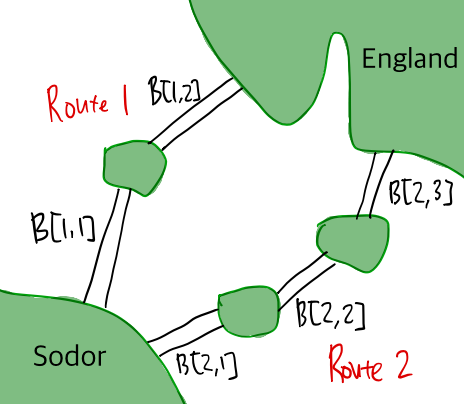시간 제한 메모리 제한 제출 정답 맞은 사람 정답 비율
1 초 512 MB 4 1 1 100.000%

## 문제

The Island of Sodor (or just Sodor) can be reached from England via bridges.

Because Sodor and England are far away, there exist multiple routes (exactly $n$ routes) that connect the two lands via one or more bridges. Specifically, route $i$ connects Sodor and England through $k_{i}$ ($k_{i} \ge 1$) bridges. Let us denote the $j$-th bridge (counting from Sodor to England) of route $i$ as $B[i, j]$. Below, we have two routes ($n = 2$) and five bridges with $k_{1} = 2$ and $k_{2} = 3$.Figure 1: Two routes and five bridges

For any two adjacent bridges of a route, $B[i, j]$ and $B[i, j+1]$ where $j+1 \le k_i$, their joint is a small island that simply serves as a connecting point of the two bridges. As demonstrated in the figure, there are exactly $n$ routes that connect Sodor and England, as the bridges do not intersect, and all joints are also distinct. In particular, if any one of the bridges of route $i$ is damaged, then route $i$ cannot be used to travel between Sodor and England.

Due to recent earthquakes in the area, some of the bridges may have experienced severe damage and become unusable. At this point we do not know exactly which bridges withstood the earthquakes and which ones were destroyed. Thanks to the inspections that were performed on the bridges prior to the incidents, for each bridge, we know the exact probability of whether it is still intact or not. Let $p[i, j]$ be the probability ($0 < p[i,j] < 1$) that bridge $B[i, j]$ is still intact after the earthquakes. Assume that the events of bridges being intact are mutually independent.

We want to know whether there is still a path between Sodor and England. However, determining whether a bridge is still intact or not is a costly operation because one needs to dispatch a large team of inspectors via choppers and boats, and therefore we want to minimize the number of inspections. Using an optimal sequence of inspections (that minimizes the expected number of inspections), what is the expected number of inspections we must perform until we are certain whether there is still a safe route between Sodor and England?

## 입력

The first line contains $n$, the number of routes ($2 \le n \le 1000$).

The following $n$ lines contain the information on each route. The $i$-th of them starts with an integer $k_i$, the number of bridges in the $i$-th route ($1 \le k_{i} \le 1000$). Then follow $k_{i}$ integers labeled as $q[i, j]$, where $1 \le j \le k_{i}$. Each $q[i, j]$ is an integer between 1 and 999, inclusive, and it means that $p[i, j] = q[i,j] / 1000$.

## 출력

Simply output the expected number of inspections for an optimal inspection sequence of the bridges. Your answer will be considered correct if its relative or absolute error is within $10^{-9}$.

## 예제 입력 1

2
3 900 900 900
2 100 100


## 예제 출력 1

3.0081


## 예제 입력 2

3
1 240
1 310
1 50


## 예제 출력 2

2.2144


## 힌트

The first input describes the example discussed in the problem description. Intuitively, route 1 is very likely to be fine, as all three bridges are expected to be intact, whereas route 2 is most likely destroyed. The optimal sequence is to inspect the three bridges of route 1 (in any order, until either route 1 is declared to be safe or damaged), and then the two bridges of route 2.

For the second input, it is optimal to inspect route 2, then route 1, and then route 3 (if needed).